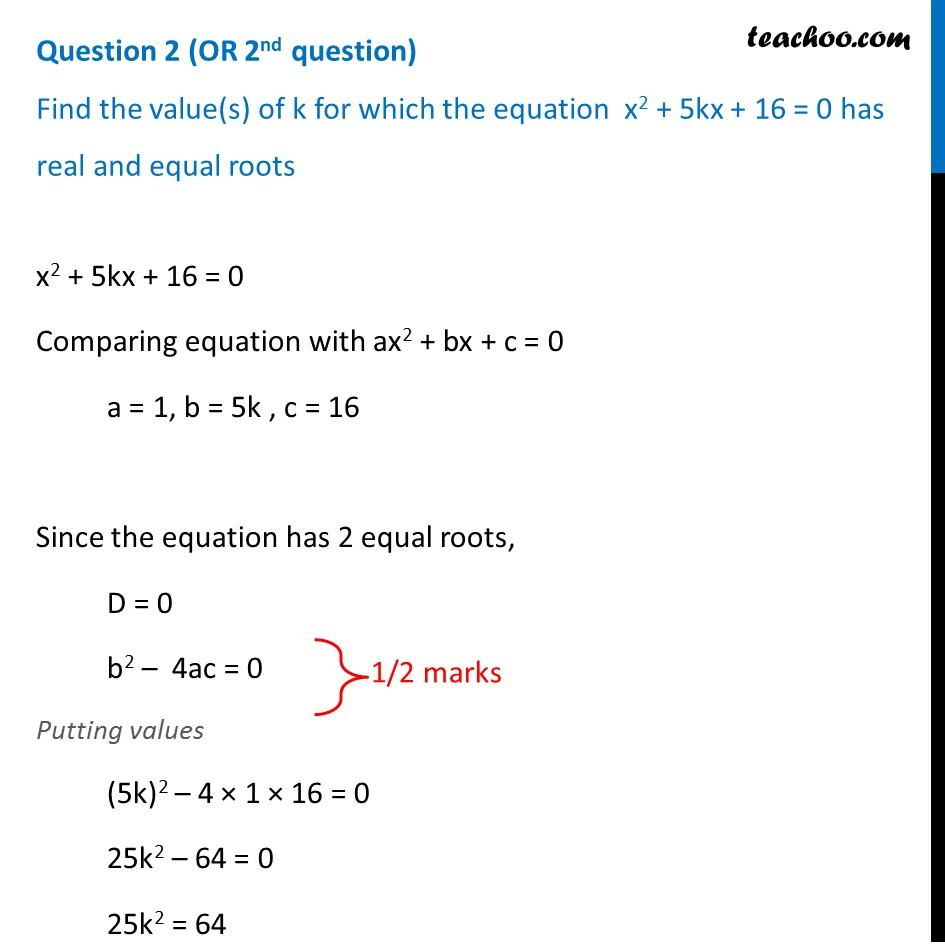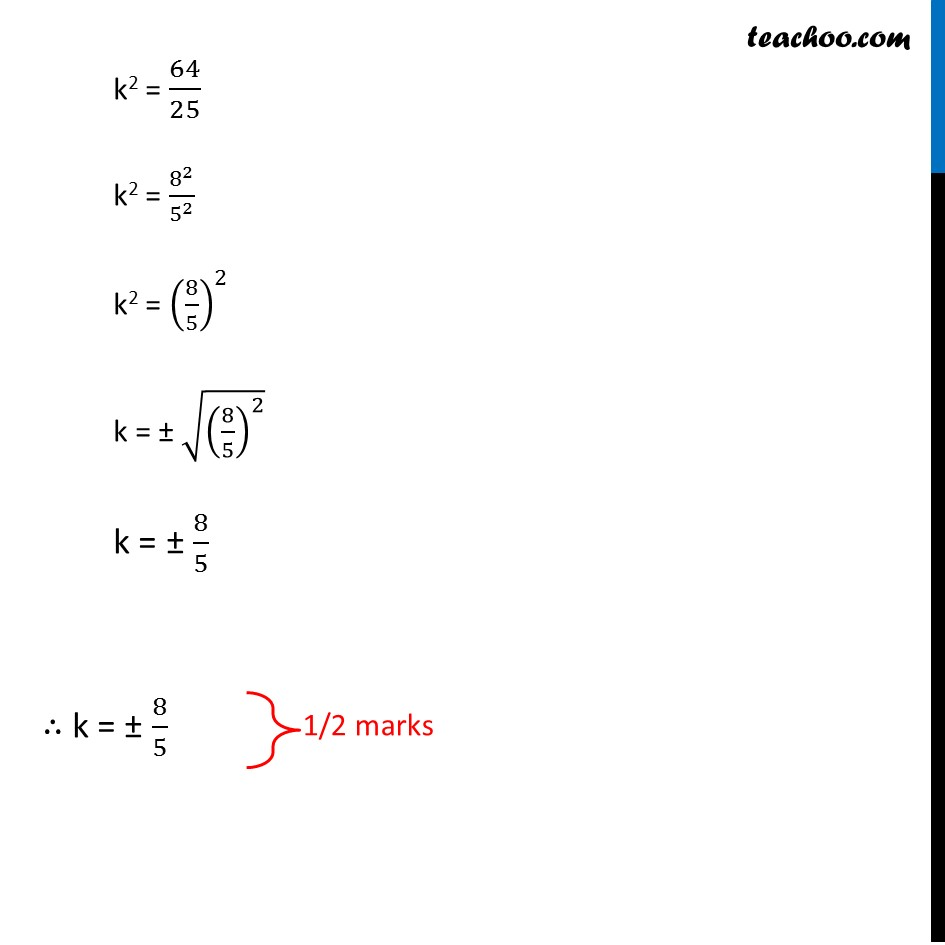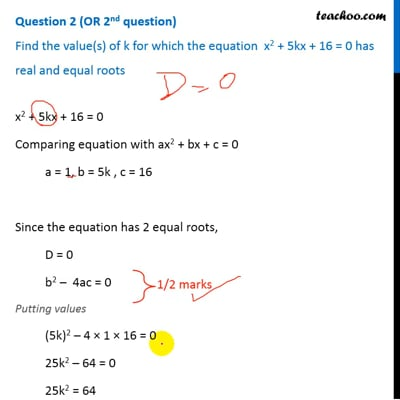CBSE Class 10 Sample Paper for 2019 Boards

Class 10
Solutions of Sample Papers for Class 10 Boards

Question 2 (OR 2 nd question)

Find the value(s) of k for which the equation x 2 + 5kx + 16 = 0 has real and equal rootsThis video is only available for Teachoo black users

Learn in your speed, with individual attention - Teachoo Maths 1-on-1 Class

### Transcript

Question 2 (OR 2nd question) Find the value(s) of k for which the equation x2 + 5kx + 16 = 0 has real and equal roots x2 + 5kx + 16 = 0 Comparing equation with ax2 + bx + c = 0 a = 1, b = 5k , c = 16 Since the equation has 2 equal roots, D = 0 b2 – 4ac = 0 Putting values (5k)2 – 4 × 1 × 16 = 0 25k2 – 64 = 0 25k2 = 64 k2 = 64/25 k2 = 8^2/5^2 k2 = (8/5)^2 k = ± √((8/5)^2 ) k = ± 8/5 ∴ k = ± 8/5